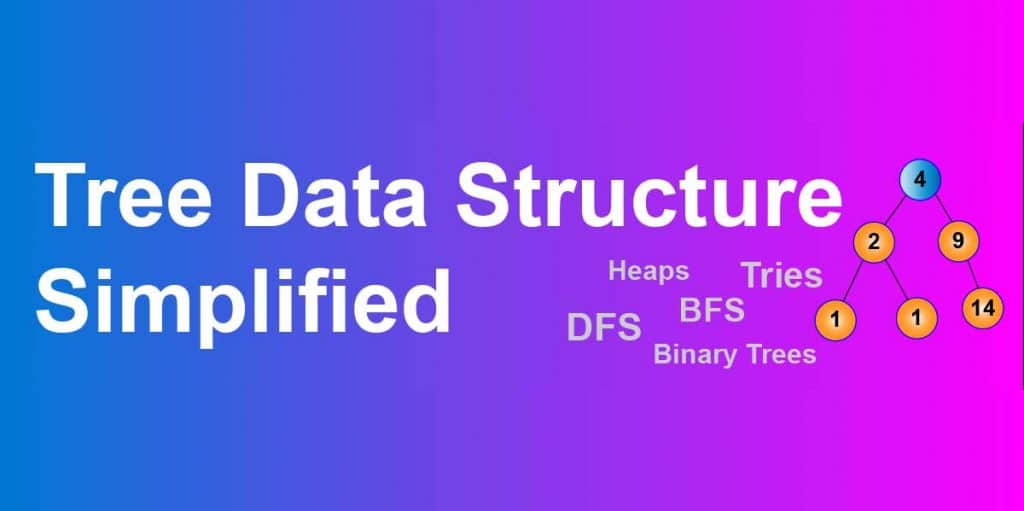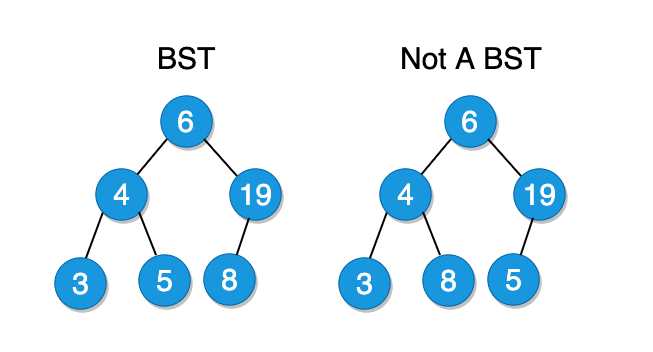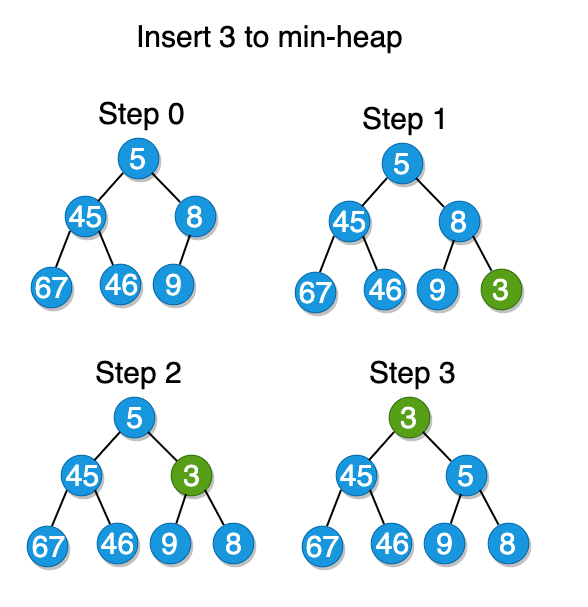# Tree Data Structure Simplified – Part 2### Outline

1. Binary search trees
2. BST Implementation
3. Binary Heaps
4. Trie

## Binary Search Tree

binary search tree is a binary tree with a unique feature – all nodes to the left of the current node must be smaller than the current node and all nodes to the right must be larger. This rule must be valid for all of the nodes in the tree, not just for the root node.In terms of performance, Binary Search Tree (BST) is a real competitor for an array. If it takes O(n) to perform an insertion/deletion operation with a sorted array, the same thing can be done in O(log \space n) with BST, if it is balanced.

It is important to note that BST is only useful when it is balanced. An unbalanced BST can be pretty slow – O(n), which defies the purpose of the data structure. There are some trees such as Red-Black Trees or AVL trees that rearrange the nodes during insertion to make sure the tree is always balanced.## BST Implementation

Let us implement the Binary Search Tree in JavaScript from scratch.

First of all, we need to define the node class for our tree. Each node needs to have three properties: data, a link to the left child, and another link to the right child. Left and right children are set to null during the Node class implementation.

// Node class
class Node {
constructor(data){
this.data = data;
this.left = null;
this.right = null;
}
}

Now we create the BST class with all the essentials functions needed to manage the data in the tree.

// BST class
class BST {
constructor(){
this.root = null;
}

// Methods to be implemented
// insert()
// remove()
}

### Insert

Insert method of the class is fairly simple. First, we create the main method, and then the helper method – insertNode. If the root node of the tree is equal to null on initial insertion, the root node will be initialized with the sent in value.

class BST {
constructor(){
this.root = null;
}
// Creates a new node and calls the insertNode method
insert(data){
const newNode = new Node(data);
if(this.root === null){
this.root = newNode;
} else {
// Finds the right spot to insert the new node
this.insertNode(this.root, newNode);
}
}

insertNode(node, newNode){
if(newNode.data < node.data && node.left){
this.insertNode(node.left, newNode);
} else if(newNode.data < node.data){
node.left = newNode;
} else if(newNode.data > node.data && node.right){
this.insertNode(node.right, newNode);
} else {
node.right = newNode;
}
}
}

### Remove

Remove method is little tricky because we have to consider the reorganization of the tree after the removal of a non-leaf node. Removing a leaf node is done by just assigning null to the parent link. But when the node to be deleted has one or two children, we have to take some additional actions.

To remove a node with one child, we set the pointer from the parent node to null.

In order to remove a node with two children, we have to do three things:

1. Find the node with the minimum value from its right branch
2. Set the node we want to delete equal to the node found in the first step
3. Set the node with minimum value to null

Here is how we implement it in code.

class BST {
constructor(){
this.root = null;
}

remove(data){
// Re-initialize the root node
this.root = this.removeNode(this.root, data);
}
removeNode(node, data){
if(node === null){
return null; // tree is empty
} else if(node.data > data){ // move left
node.left = this.removeNode(node.left, data);
return node;
} else if(node.data < data){ // move right
node.right = this.removeNode(node.right, data);
return node;
} else {
// Delete a leaf node
if(!node.left && !node.right){
return null;
}

// Delete a node with 1 child
if(!node.left){
return node.right;
} else if(!node.right){
return node.left;
}

// Delete a node with 2 children
const min = this.findMinimumValueNode(node.right);
node.data = min.data;

node.right = this.removeNode(node.right, min.data);
return node;
}
}
findMinimumValueNode(node){
// if left node is null, then this node is the minimum
// if not, we recursively find the minimum
return !node.left ? node : this.findMinimumValueNode(node.left);
}
inOrderPrint(node){
if(node !== null){
this.preOrder(node.left);
console.log(node.data);
this.preOrder(node.right);
}
}

getRoot(){
return this.root;
}
}

Now we can use methods to create and manage a binary search tree.

const Tree = new BST();

Tree.insert(30);
Tree.insert(9);
Tree.insert(100);
Tree.insert(45);
Tree.insert(166);

Tree.inOrderPrint(Tree.getRoot());
/*
30
/  \
9	  100
/ \
45  166

Print: 9 30 45 100 166
*/

Tree.remove(100);
Tree.inOrderPrint(Tree.getRoot());
/*
30
/  \
9	  45
\
166

Print: 9 30 45 166
*/

## Binary Heaps

Binary heaps are just binary trees with unique features. There are two type of Binary Heaps – Min-Heaps and Max-Heaps.

### Min-Heaps

Min-Heap is a binary tree which must have the following qualities:

1. It should be a complete binary tree. Meaning the tree should be filled except for the last rightmost branch.
2. Each node must be smaller than its children.
3. Root must be the minimum element in the tree.

### Max-Heaps

Max-Heaps are almost the same as min-heaps except for the elements are in descending order instead of ascending order like in min-heaps.

There are two main methods used with min-heaps: insert and extract_minimum.

#### Insert

To insert a value into a min-heap, we place the new node at the rightmost bottom of the tree. That way we can always make sure that the tree stays complete. Then we need to re-organize the tree to bring the node with the minimum value to the top. The whole process takes about O(log n) to execute.#### Extracting the minimum value

Extracting the minimum value from the min-heap is simple because the root node always holds the minimum element. The only thing to consider is the time when we need to remove the root node from the tree. In that case, we first remove the minimum element and then set the root equal to the rightmost bottom node. If after the swap the min-heap is out of order, we keep swapping the root node with its children until it is restored.### Heaps vs. Arrays

Heaps can also be stored as arrays. Add a new element to a tree is equivalent of pushing to the back of the array. Traversing the tree when it is an array can be little tricky though. Because it is difficult to know which element is the right child or left child of a node. Therefore, we need to use some indexing technique to accomplish this task. There are various formulas for indexing, but the following is used more often and easy to remember.

Left child: A node at index i has its left child at index 2 * i + 1 That means, node at index 0 would have its left child at index 2 * 0 + 1 => 1

Right child: A node at index i has its left child at index 2 * i + 2 Meaning right child comes after the left child.

Parent: A node’s parent node is located at index (i – 1)/2.

## Tries

Trie, also called as prefix tree or radix tree, is another type of tree with a distinctive feature – it is designed to efficiently store and retrieve strings. In a trie, we store characters in each node, and each branch down the tree resembles a word.

Here is an example of a trie that stores “Simon”, “Simba” and “Lisa”:

A trie can save a lot of space when implemented correctly. As we can see from the trie above, the words Simon and Simba have the common prefix of “Sim”. So we store that prefix once and use it multiple times.

Tries are especially useful for predicting the possible words given some prefixes. For example, providing suggestions when we are trying to search for a state in a form. We type in “New ” in the form and it shows us what states are available in the dictionary that starts with the word “New “: “New York,” “New Mexico,” “New Hampshire,” etc.

Each node in a trie may have up to 26 children (English alphabet). And there must be a way of identifying the endings of words. Usually, the endings are indicated by adding a special character node to every word. Let’s say we add a node that contains a hashtag character “#” at the end of each valid word.In the worst case scenario, it can take up to O(k) runtime to perform an insertion or a lookup in a trie. k being the number of nodes. Space complexity can be as bad as O(n*k). However, a trie is a great data structure to implement with applications that heavily rely on prefixes. It is even better than hash tables in this regard because hash tables cannot tell us if a string is a prefix of any word or not.